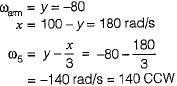Courses

# Test: Gear Trains - 2

## 10 Questions MCQ Test Topicwise Question Bank for Mechanical Engineering | Test: Gear Trains - 2

Description
This mock test of Test: Gear Trains - 2 for Mechanical Engineering helps you for every Mechanical Engineering entrance exam. This contains 10 Multiple Choice Questions for Mechanical Engineering Test: Gear Trains - 2 (mcq) to study with solutions a complete question bank. The solved questions answers in this Test: Gear Trains - 2 quiz give you a good mix of easy questions and tough questions. Mechanical Engineering students definitely take this Test: Gear Trains - 2 exercise for a better result in the exam. You can find other Test: Gear Trains - 2 extra questions, long questions & short questions for Mechanical Engineering on EduRev as well by searching above.
QUESTION: 1

### A gear box in an automobile utilizes

Solution:

In auto mobile gear box sun and planet or epicyclic gear train is used.

QUESTION: 2

### Two parallel shafts whose axes are separated by a distance of 75 mm are to be connected a spur gear set so that the output shaft rotates at 50% of the speed of the input shaft which of the following could be the possible pitch circle diameters of the gears.

Solution:

Centre distance between the shaft = 75 mm
Hence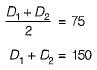Also,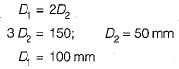QUESTION: 3

### Consider the following specifications of gears A, B, C and D: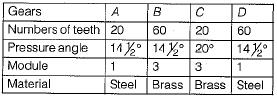Which of these gears form the pair of spur gears to achieve a gear ratio of 3?

Solution:

Combination of gear B-C and C-D can not use because pressure angle is not same.
Similarly combination of gear A-B can not use because module is not some hence combination of A-D should be taken because they have some module and pressure angle with gear ratio of 3.

QUESTION: 4

A pinion and gear are in mesh with each other. The gear ratio is 2 and the moment of inertias of the pinion and the gear are 3 kg m2 and 5 kg m2 respectively. For the pinion to have an angular acceleration of 4 rad/s2, the torque to be applied to the pinion shaft is

Solution: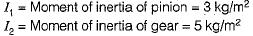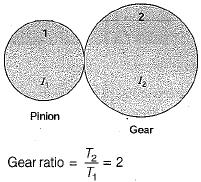⇒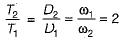Since the power transmission is same,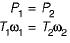⇒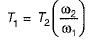But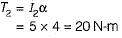⇒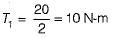QUESTION: 5

The sun gear in the figure is driven clockwise at 100 rpm. The ring gear is held stationary. For the number of teeth shown on the gears, the arm rotates at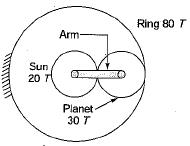Solution: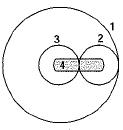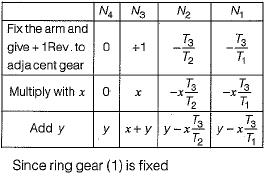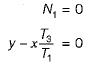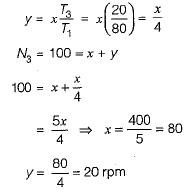QUESTION: 6

In the epicyclic gear train shown in the figure, TA = 40, TB = 20. For three revolutions of the arm, the gear B will rotale through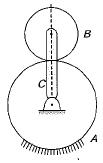Solution: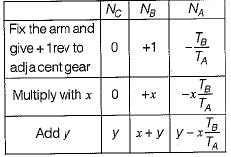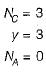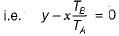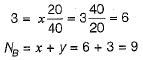QUESTION: 7

In an epicyclic gear train with a sun gear, planet gear and a moving arm. The ratio of number of teeth of the sun gear to that of the planet gear is 3. If the planet gear is fixed and the arm has an angular velocity of 150 RPM the sun gear will rotate with

Solution: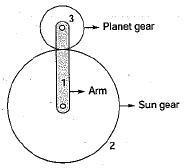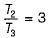If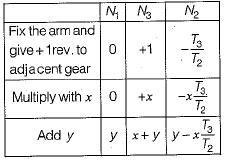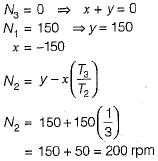QUESTION: 8

Match List-l with List-ll and select the correct answer using the codes given below
List-l
A. Compound gear train
B. Epicyclic spur gear train with brake bands
C. Worm and worm-wheel gear train
D. Epicyclic bevel gear train
List-ll
1. Automobile gear box
2. Automatic transmission of automobile
3. Speed reducers for lifts
4. Automobile differential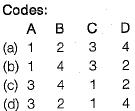Solution:
QUESTION: 9

100 kW power is supplied to the machine through a gear box which uses an epicyclic gear train. The power is supplied at 100 rad/s. The speed of the output shaft of the gear box is 10 rad/s in a sense opposite to the input speed. What is the holding toque on the fixed gear of the train?

Solution:

Holding torque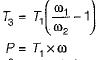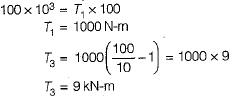QUESTION: 10

For the epicyclic gear arrangement shown in the figure, ω2 = 100 rad/s clockwise (CW) and ωarm = 80 rad/s counter clockwise (CCW). The angular velocity ω5 (in rad/s) is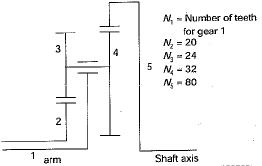Solution:

Given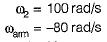teeth denoted by N,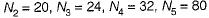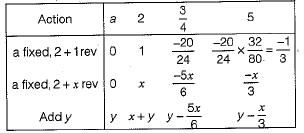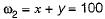and